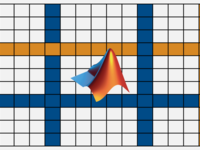# MATLAB Fundamentals - Matrices and Arrays

Curated by : Matt Tearle

Average Problem Difficulty:Community Group Solver

+50 points

Problem Title Likes Solvers Difficulty

Created by: Renee Coetsee

7

296

#### Problem 55230. Find Closest Constant

Created by: Renee Coetsee

Tags math, vector, matrix

4

229

#### Problem 55240. Calculate the mean of each half of a matrix

Created by: Matt Tearle

Tags matrix

1

215

#### Problem 55250. Find the minimum of the column-maximums of a matrix

Created by: Matt Tearle

2

213

#### Problem 55225. Simpson's Paradox - Calculate correlation coefficients for groups of data

Created by: Matt Tearle

4

129

6

400

#### Problem 54485. Dog Statistics

Created by: Ben Placek

Tags statistics

3

127

#### Problem 55235. Determine if x is a combination of m and n

Created by: Renee Coetsee

Tags math, vector, matrix

6

134

#### Problem 55245. Calculating Student Grades

Created by: Renee Coetsee

Tags matrix

6

142

#### Problem 54440. Create an arrow matrix

Created by: Ben Placek

2

189

#### Recent Activity

 on 29 May 2023 at 7:58 on 29 May 2023 at 7:37 on 29 May 2023 at 7:20 on 29 May 2023 at 6:59 on 29 May 2023 at 6:47

### Community Treasure Hunt

Find the treasures in MATLAB Central and discover how the community can help you!

Start Hunting!